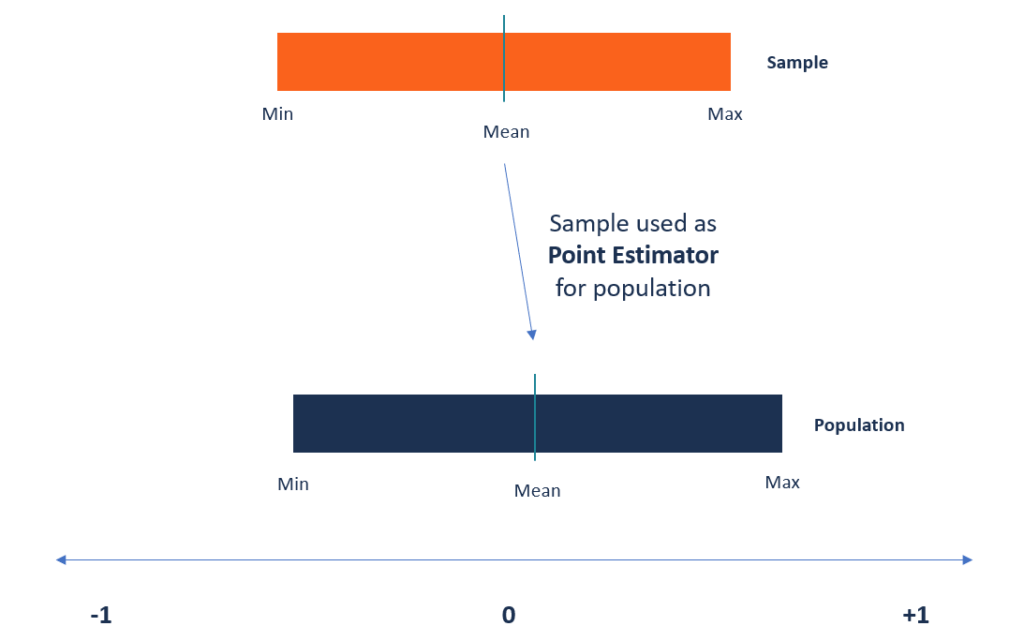# Point Estimators

A function that is used to find an approximate value of a population parameter from random samples of the population

## What are Point Estimators?

Point estimators are functions that are used to find an approximate value of a population parameter from random samples of the population. They use the sample data of a population to calculate a point estimate or a statistic that serves as the best estimate of an unknown parameter of a population.Most often, the existing methods of finding the parameters of large populations are unrealistic. For example, when finding the average age of kids attending kindergarten, it will be impossible to collect the exact age of every kindergarten kid in the world. Instead, a statistician can use the point estimator to make an estimate of the population parameter.

### Properties of Point Estimators

The following are the main characteristics of point estimators:

#### 1. Bias

The bias of a point estimator is defined as the difference between the expected value of the estimator and the value of the parameter being estimated. When the estimated value shows zero bias, it is considered to be unbiased, while in instances when the estimated value of the parameter and the value of the parameter being estimated are equal, the estimator is considered biased.

Also, the closer the expected value of a parameter is to the value of the parameter being measured, the lesser the bias is.

#### 2. Consistency

Consistency tells us how close the point estimator stays to the value of the parameter as it increases in size. The point estimator requires a large sample size for it to be more consistent and accurate. You can also check if a point estimator is consistent by looking at its corresponding expected value and variance. For the point estimator to be consistent, the expected value should move towards the true value of the parameter.

#### 3. Most efficient or unbiased

The most efficient point estimator is the one with the smallest variance of all the unbiased and consistent estimators. The variance measures the level of dispersion from the estimate, and the smallest variance should vary the least from one sample to the other.

Generally, the efficiency of the estimator depends on the distribution of the population. For example, in a normal distribution, the mean is considered more efficient than the median, but the same does not apply in asymmetrical distributions.

### Point Estimation vs. Interval Estimation

The two main types of estimators in statistics are point estimators and interval estimators. Point estimation is the opposite of interval estimation. It produces a single value while the latter produces a range of values. A point estimator is a statistic used to estimate the value of an unknown parameter of a population. It uses sample data when calculating a single statistic that will be the best estimate of the unknown parameter of the population.

On the other hand, interval estimation uses sample data to calculate the interval of the possible values of an unknown parameter of a population. The interval of the parameter is selected in a way that it falls within a 95% or higher probability, also known as the confidence interval. The confidence interval is used to indicate how reliable an estimate is, and it is calculated from the observed data. The endpoints of the intervals are referred to as the upper and lower confidence limits.

### Common Methods of Finding Point Estimates

The process of point estimation involves utilizing the value of a statistic that is obtained from sample data to get the best estimate of the corresponding unknown parameter of the population. Several methods can be used to calculate the point estimators, and each method comes with different properties.

#### 1. Method of moments

The method of moments of estimating parameters was introduced in 1887 by Russian mathematician Pafnuty Chebyshev. It starts by taking known facts about a population and then applying the facts to a sample of the population. The first step is to derive equations that relate the population moments to the unknown parameters.

The next step is to draw a sample of the population to be used to estimate the population moments. The equations derived in step one are then solved using the sample mean of the population moments. It produces the best estimate of the unknown population parameters.

#### 2. Maximum likelihood estimator

The maximum likelihood estimator method of point estimation attempts to find the unknown parameters that maximize the likelihood function. It takes a known model and uses the values to compare data sets and find the most suitable match for the data.

For example, a researcher may be interested in knowing the average weight of babies born prematurely. Since it would be impossible to measure all babies born prematurely in the population, the researcher can take a sample from one location. Since the weight of pre-term babies follows a normal distribution, the researcher can use the maximum likelihood estimator to find the average weight of the entire population of pre-term babies based on the sample data.

### More Resources

CFI is the official provider of the Financial Modeling and Valuation Analyst (FMVA)™ certification program, designed to transform anyone into a world-class financial analyst.

To keep learning and developing your knowledge of financial analysis, we highly recommend the additional resources below:

• Basic Statistics Concepts for Finance
• Hypothesis Testing
• Independent Events
• P-value

### Financial Analyst Certification

Become a certified Financial Modeling and Valuation Analyst (FMVA)® by completing CFI’s online financial modeling classes and training program!